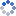加载中…MATLAB技术论坛
• 博客等级：• 博客积分：0
• 博客访问：590,608
• 关注人气：489
• 获赠金笔：0支
• 赠出金笔：0支
• 荣誉徽章：

## 【原创】基于Householder变换QR分解Matlab代码

(2010-01-17 22:52:38)

### 杂谈

Wish my dear girl，healthy pretty and oveliness

2010-1-17 22:53:19

function [Q,R]=qrhs(A)
% 基于Householder变换，将方阵A分解为A=QR，其中Q为正交矩阵，R为上三角阵
%
% 参数说明
% A：需要进行QR分解的方阵
% Q：分解得到的正交矩阵
% R：分解得到的上三角阵
%
% 实例说明
% A=[-12 3 3;3 1 -2;3 -2 7];
% [Q,R]=qr(A) % 调用MATLAB自带的QR分解函数进行验证
% [q,r]=qrhs(A) % 调用本函数进行QR分解
% q*r-A % 验证 A=QR
% q'*q % 验证q的正交性
% norm(q) % 验证q的标准化，即二范数等于1
%
% 线性代数基础知识
% 1.B=P*A*inv(P)，称A与B相似，相似矩阵具有相同的特征值
% 2.Q*Q'=I，称Q为正交矩阵，正交矩阵的乘积仍为正交矩阵
%
%
% by dynamic of Matlab技术论坛
% contact me matlabsky@gmail.com
% 2010-01-17 22:49:51
%
n=size(A,1);
R=A;
Q=eye(n);
for i=1:n-1
x=R(i:n,i);
y=[1;zeros(n-i,1)];
Ht=householder(x,y);
H=blkdiag(eye(i-1),Ht);
Q=Q*H;
R=H*R;
end

function [H,rho]=householder(x,y)
% 求解正交对称的Householder矩阵H，使Hx=rho*y，其中rho=-sign(x(1))*||x||/||y||
%
% 参数说明
% x：列向量
% y：列向量，x和y必须具有相同的维数
%
% 实例说明
% x=[3 5 6 8]';
% y=[1 0 0 0]';
% [H,rho]=householder(x,y);
% H*x-rho*y % 验证Hx=rho*y
% H'*H % 验证正交
%
% 关于HouseHolder变换
% 1.H=I-2vv'，其中||v||=1，我们称H为反射(HouseHolder)矩阵，易证H对称且正交
% 2.如果||x||=||y||，那么存在HouseHolder矩阵H=I-2vv，其中v=±(x-y)/||x-y||，使Hx=y
% 3.如果||x||≠||y||，那么存在HouseHolder矩阵H，使Hx=rho*y，其中rho=-sign(x(1))*||x||/||y||
% 4.Householder矩阵，常用于将一个矩阵A通过正交变换对角阵
%
x=x(:);
y=y(:);
if length(x)~=length(y)
error('The Column Vectors X and Y Must Have The Same Length!');
end
rho=-sign(x(1))*norm(x)/norm(y);
y=rho*y;
v=(x-y)/norm(x-y);
I=eye(length(x));
H=I-2*v*v';

0